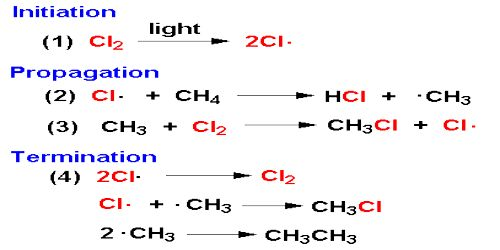# Hydrogen-Chlorine Chain Reaction

Hydrogen-Chlorine Chain Reaction

An example of chain reaction is the reaction between hydrogen and chlorine the presence of light. According to the laws of photochemistry, one quantum of radiation is absorbed by one molecule of the reactant producing two atoms of chlorine. In the case of hydrogen-chlorine reaction it was found that as many as 106 molecules of HCl are produced for each quantum of radiation absorbed. Such a large yield of HCl as a result of the breakup of only one molecule of chlorine was explained using the following steps:

1. Cl2 + light → Cl* + Cl*
2. Cl* + H2 → HCl + H*
3. H* + Cl2 → HCl + Cl*
4. Cl* + H2 → HCl + H* etc.

The first step in the reaction is the absorption of radiation by chlorine molecules resulting in the production of two chlorine atoms, which are very reactive. In step 2 is shown the reaction between a chlorine atom and a hydrogen molecule when a hydrogen atom is produced. This, in turn, reacts with a molecule of chlorine to produce one molecule of hydrogen chloride and one atom of chlorine. This chlorine atom reacts again and the cycle is repeated. A reaction which was started with the formation of two chlorine atoms has now become self-propagating and a chain reaction takes place.It would seem from this discussion that the reaction will continue to proceed in this manner till all the reactants are consumed. Such, however, is not the case as one or more of the following reactions-

1. Cl* + Cl* → Cl2
2. H* + H* → H2
3. H* + Cl* → HCl

may terminate the self-propagating steps of the chain. Such reactions are usually known to occur in the presence of a third body, usually the wall of the container, which can take away the energy liberated when the atoms combine. Step 1 is known as the initiation step as the chain starts as a result of this reaction. Steps 2 to 4 are the chain propagating steps while steps 5 to 7 are the terminating steps, as the chain of production of chlorine atom is blocked by these reactions.